This site uses cookies. By continuing to browse the ConceptDraw site you are agreeing to our Use of Site Cookies.
ConceptDraw Samples | Graphs and charts — Divided bar diagram

# Divided Bar Diagrams

Samples of divided bar charts are created using ConceptDraw DIAGRAM diagramming and vector drawing software and the Divided Bar Diagrams solution.

ConceptDraw DIAGRAM provides export of vector graphic multipage documents into multiple file formats: vector graphics (SVG, EMF, EPS), bitmap graphics (PNG, JPEG, GIF, BMP, TIFF), web documents (HTML, PDF), PowerPoint presentations (PPT), Adobe Flash (SWF).

## Tutorials and Solutions:

Video Tutorials: ConceptDraw Solution Park

Solutions: Divided Bar Diagrams for ConceptDraw DIAGRAM

## Sample 1: Global Natural Gas Consumption

Divided Bar Diagrams sample: Global natural gas consumption.

This example is created using ConceptDraw DIAGRAM diagramming software enhanced with Divided Bar Diagrams solution from ConceptDraw Solution Park.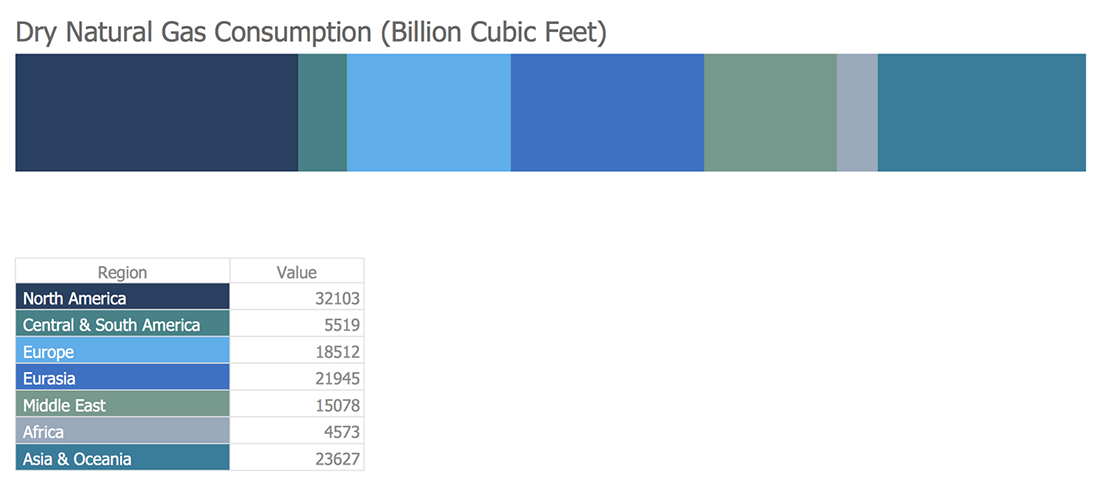## Sample 2: Composition of State Government Revenue

Divided Bar Diagrams sample: Composition of state government revenue.

This example is created using ConceptDraw DIAGRAM diagramming software enhanced with Divided Bar Diagrams solution from ConceptDraw Solution Park.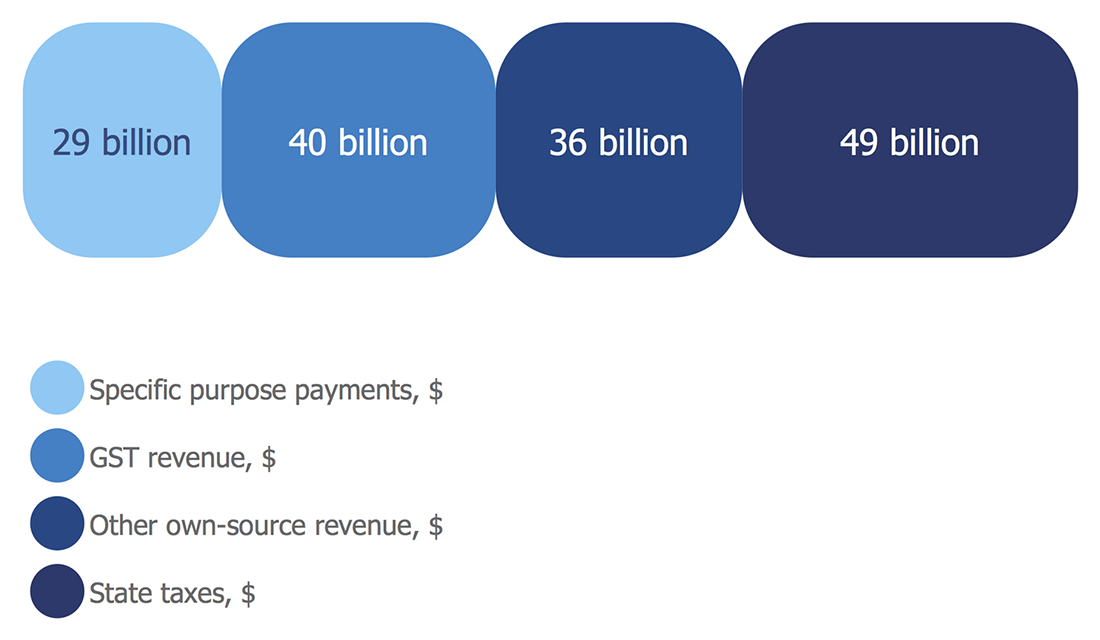## Sample 3: Composition of Estates

Divided Bar Diagrams sample: Composition of estates.

This example is created using ConceptDraw DIAGRAM diagramming software enhanced with Divided Bar Diagrams solution from ConceptDraw Solution Park.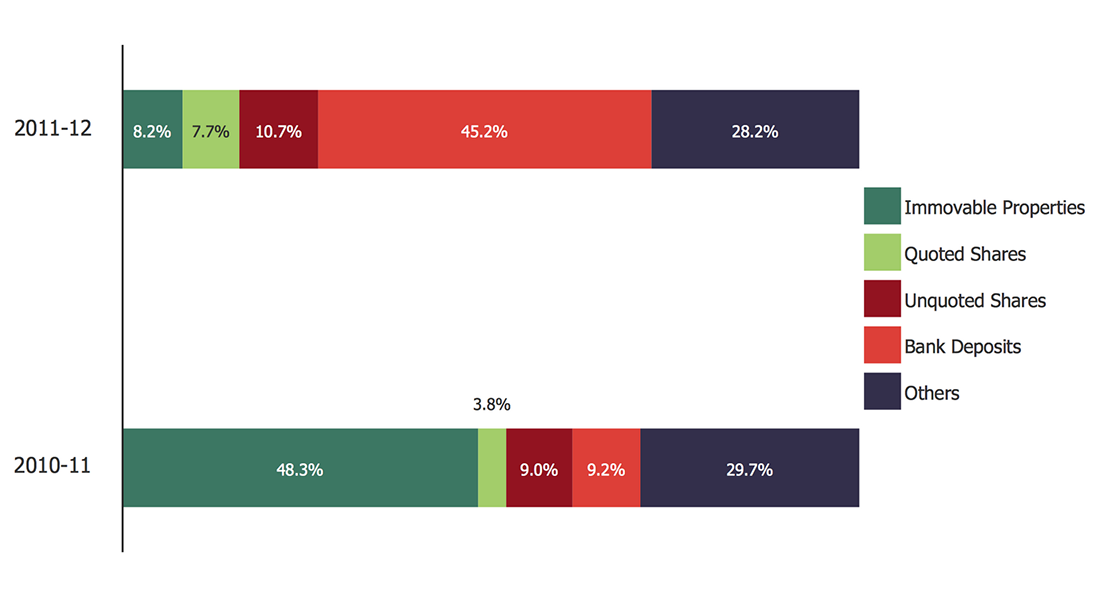## Sample 4: London Election Results

Divided Bar Diagrams sample: London election results.

This example is created using ConceptDraw DIAGRAM diagramming software enhanced with Divided Bar Diagrams solution from ConceptDraw Solution Park.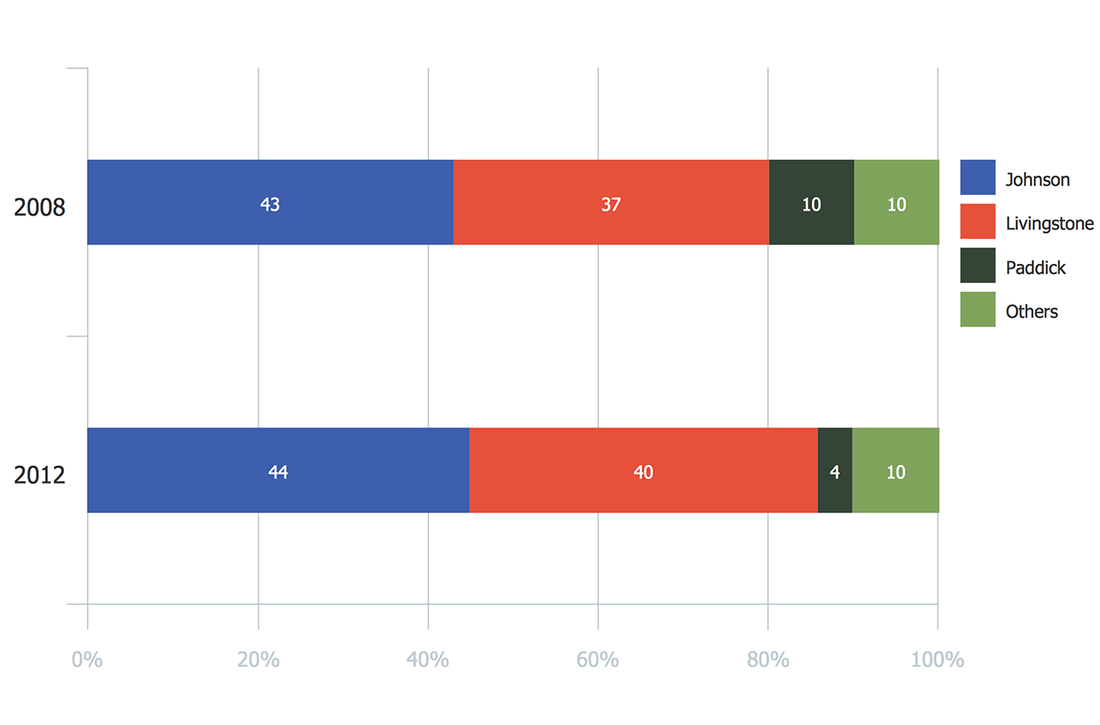## Sample 5: Petroleum Products Yielded from One Barrel of Crude Oil

Divided Bar Diagrams sample: Petroleum products yielded from one barrel of crude oil.

This example is created using ConceptDraw DIAGRAM diagramming software enhanced with Divided Bar Diagrams solution from ConceptDraw Solution Park.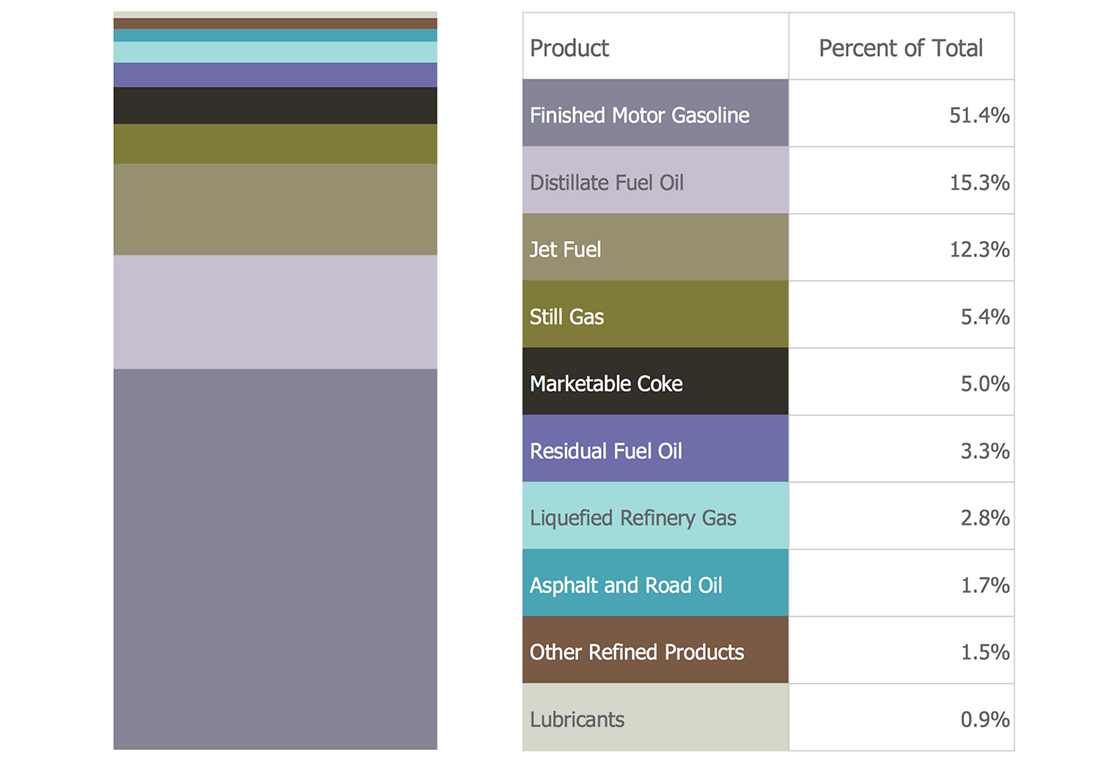## Sample 6: Shares of Aggregate Income

Divided Bar Diagrams sample: Shares of Aggregate Income.

This example is created using ConceptDraw DIAGRAM diagramming software enhanced with Divided Bar Diagrams solution from ConceptDraw Solution Park.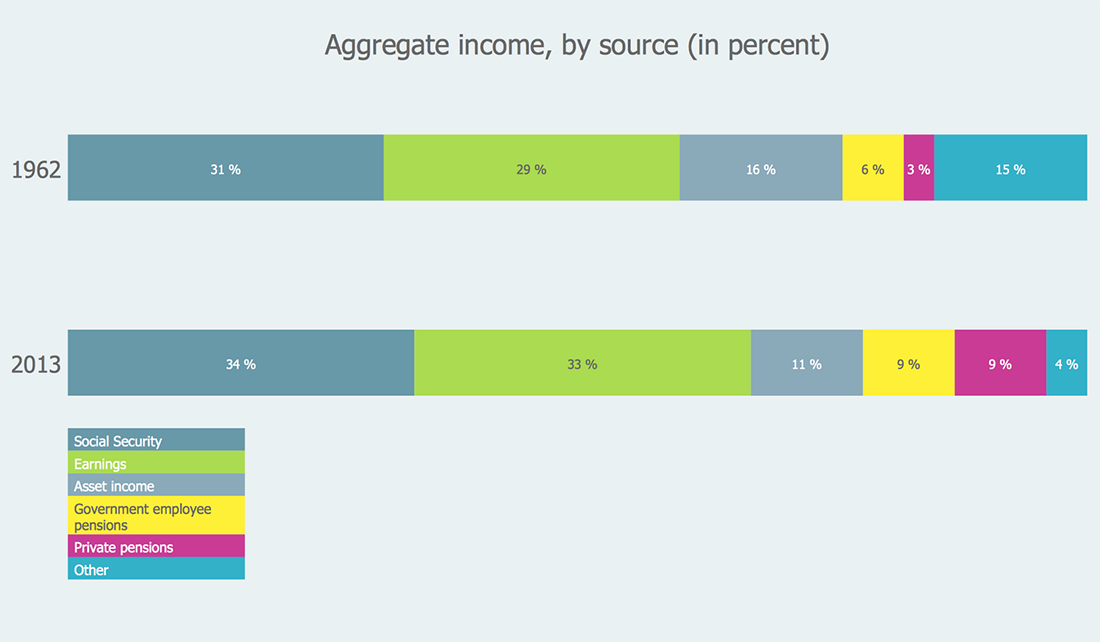## Sample 7: Taking Up Flexible Work Options Leave Arrangements

Divided Bar Diagrams sample: Taking Up Flexible Work Options Leave Arrangements.

This example is created using ConceptDraw DIAGRAM diagramming software enhanced with Divided Bar Diagrams solution from ConceptDraw Solution Park.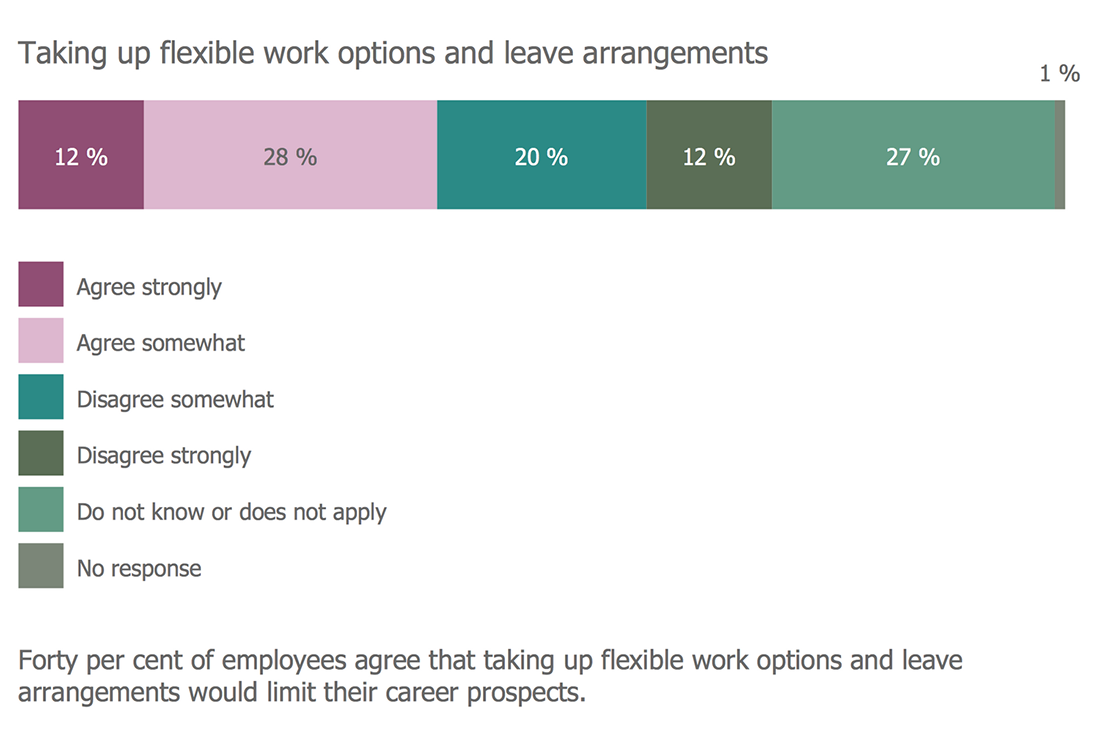## Sample 8: U.S. Primary Energy Consumption by Source and Sector

Divided Bar Diagrams sample: U.S. Primary Energy Consumption by Source and Sector.

This example is created using ConceptDraw DIAGRAM diagramming software enhanced with Divided Bar Diagrams solution from ConceptDraw Solution Park.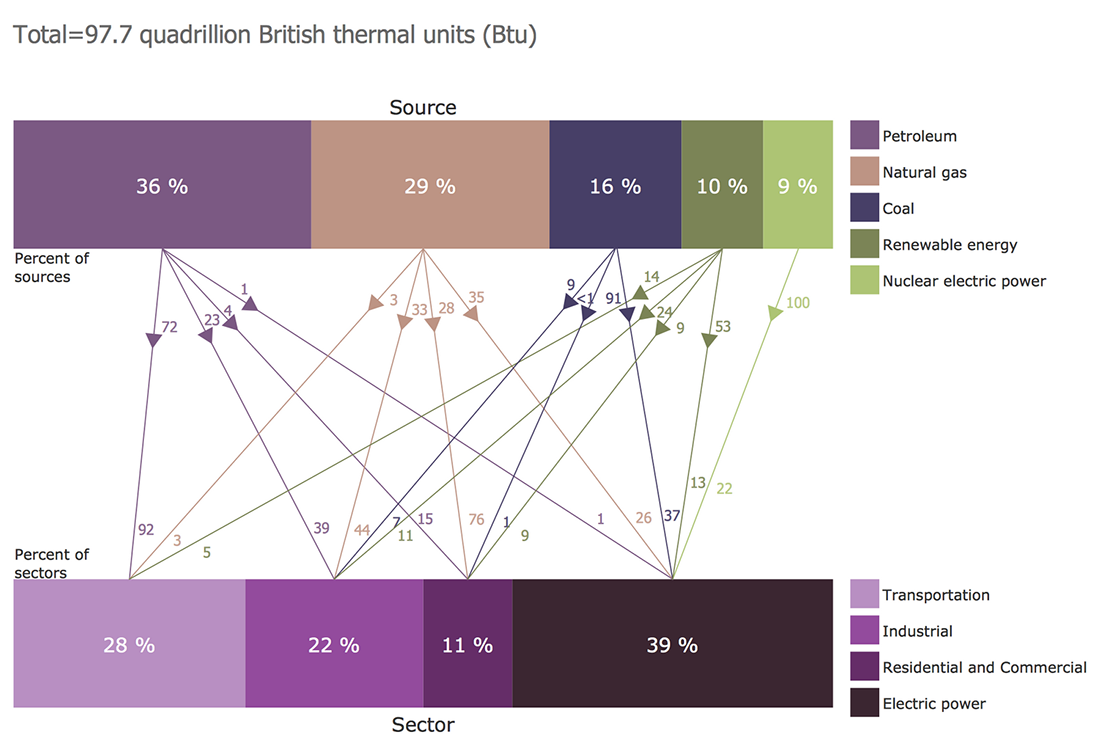## Sample 9: Workplace Culture

Divided Bar Diagrams sample: Workplace Culture.

This example is created using ConceptDraw DIAGRAM diagramming software enhanced with Divided Bar Diagrams solution from ConceptDraw Solution Park.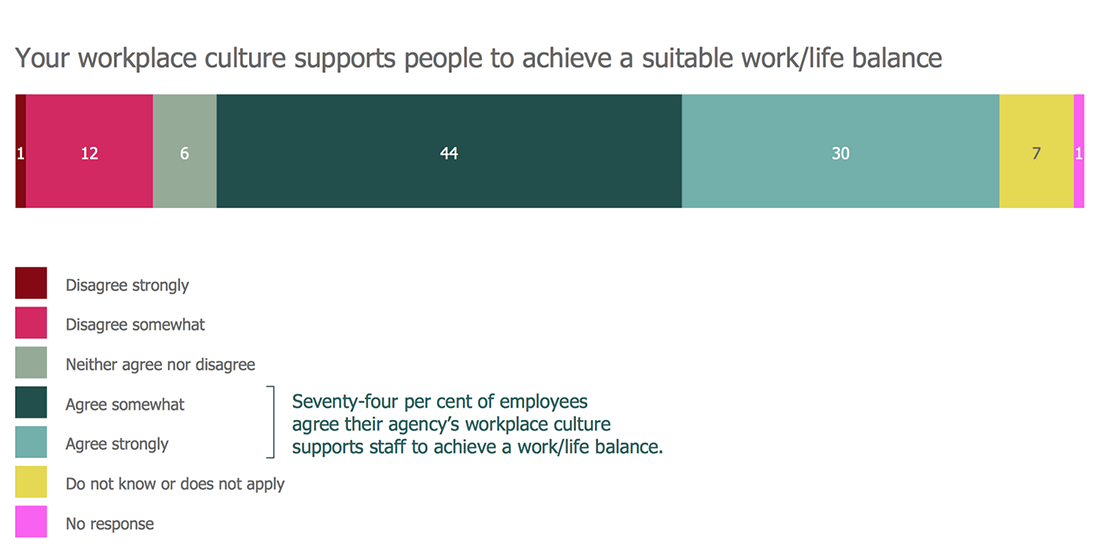## Sample 10: Your Agency is Committed to Creating a Diverse Workforce

Divided Bar Diagrams sample: Your Agency is Committed to Creating a Diverse Workforce.

This example is created using ConceptDraw DIAGRAM diagramming software enhanced with Divided Bar Diagrams solution from ConceptDraw Solution Park.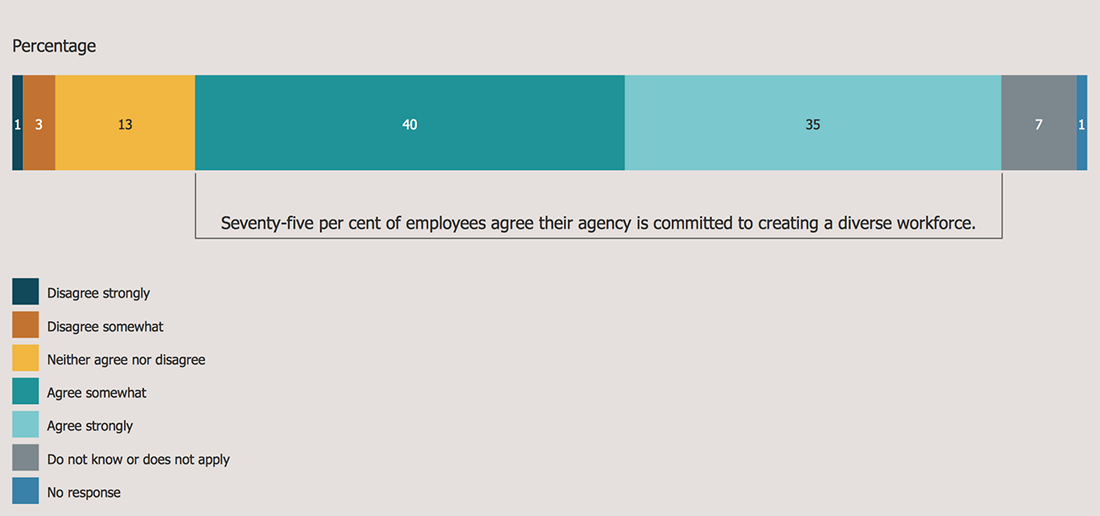All samples are copyrighted CS Odessa's.

Usage of them is covered by Creative Commons “Attribution Non-Commercial No Derivatives” License.

The text you can find at: https://creativecommons.org/licenses/by-nc-nd/3.0